• 2018-11-13 18:53:46

# 时间序列分析需要解决的问题

假设我们有这么一段数据（采样自移动公司某段时间的开户客户数目）
下载地址，包含本实验用到的数据/资料以及全部代码，实验报告，引用请告知，关注并留言获取提取码࿰去趋势
更多相关内容
• Datawhale干货译者：陈超，北京大学，数据派THU 本文约7500字，建议阅读20+分钟本文介绍了时间序列的定义、特征并结合实例给出了时间序列在Python中评价指标和方法。...

Datawhale干货

译者：陈超北京大学，数据派THU



本文约7500字，建议阅读20+分钟本文介绍了时间序列的定义、特征并结合实例给出了时间序列在Python中评价指标和方法。


时间序列是在规律性时间间隔上记录的观测值序列。本指南将带你了解在Python中分析给定时间序列的特征的全过程。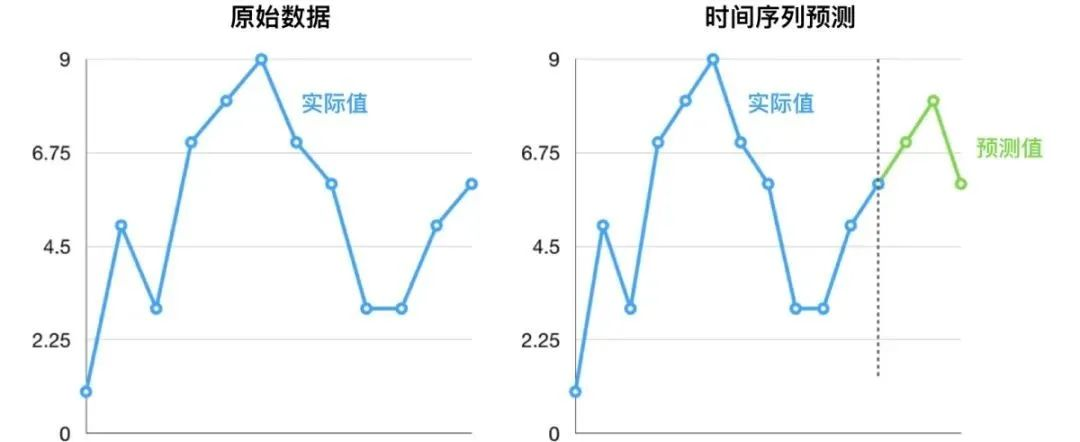主要内容

1. 什么是时间序列？

2. 如何在Python中导入时间序列？

3. 什么是面板数据？

4. 时间序列可视化

5. 时间序列的模式

6. 时间序列的加法和乘法

7. 如何将时间序列分解？

8. 平稳和非平稳时间序列

9. 如何获取平稳的时间序列？

10. 如何检验平稳性？

11. 白噪音和平稳序列的差异是什么？

12. 如何去除时间序列的线性分量？

13. 如何消除时间序列的季节性？

14. 如何检验时间序列的季节性？

15. 如何处理时间序列中的缺失值？

16. 什么是自回归和偏自回归函数？

17. 如何计算偏自回归函数？

18. 滞后图

19. 如何估计时间序列的预测能力？

20. 为什么以及怎样使时间序列平滑？

21. 如何使用Granger因果检验来获知时间序列是否对预测另一个序列帮助？

22. 下一步是什么?

1. 什么是时间序列?

时间序列是在规律性时间间隔记录的观测值序列。

依赖于观测值的频率，典型的时间序列可分为每小时、每天、每周、每月、每季度和每年为单位记录。有时，你可能也会用到以秒或者分钟为单位的时间序列，比如，每分钟用户点击量和访问量等等。

1.1 为什么要分析时间序列呢？

因为它是你做序列预测前的一步准备过程。而且，时间序列预测拥有巨大的商业重要性，因为对商业来说非常重要的需求和销量、网站访问人数、股价等都是时间序列数据。

1.2 所以时间序列分析包括什么内容呢？

时间序列分析包括理解序列内在本质的多个方面以便于你可更好地了解如何做出有意义并且精确的预测。

## 2. 如何在Python中导入时间序列？

所以怎样导入时间序列数据呢？典型的时间序列数据以.csv格式或者其他表格形式存储，包括两列：日期和测量值。

from dateutil.parser import parse
import matplotlib as mpl
import matplotlib.pyplot as plt
import seaborn as sns
import numpy as np
import pandas as pd
plt.rcParams.update({'figure.figsize': (10, 7), 'figure.dpi': 120})

# Import as Dataframe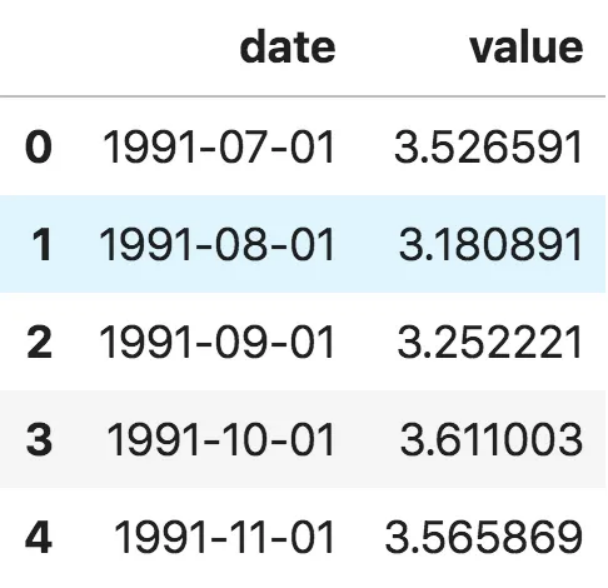数据框时间序列

ser = pd.read_csv('https://raw.githubusercontent.com/selva86/datasets/master/a10.csv', parse_dates=['date'], index_col='date')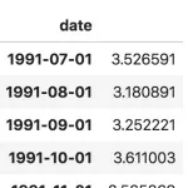时间序列

注意，在此序列当中，‘value’列的位置高于date以表明它是一个序列。

3. 什么是面板数据？

面板数据也是基于时间的数据集。

差异在于，除了时间序列，它也包括同时测量的一个或多个相关变量。

通常来看，面板数据当中的列包括了有助于预测Y的解释型变量，假设这些列将在未来预测阶段有用。

面板数据的例子如下：

# dataset source: https://github.com/rouseguy
df = df.loc[df.market=='MUMBAI', :]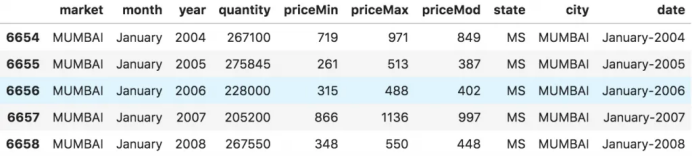面板数据

## 4. 时间序列可视化

让我们用matplotlib来对序列进行可视化。

# Time series data source: fpp pacakge in R.
import matplotlib.pyplot as plt

# Draw Plot
def plot_df(df, x, y, title="", xlabel='Date', ylabel='Value', dpi=100):
plt.figure(figsize=(16,5), dpi=dpi)
plt.plot(x, y, color='tab:red')
plt.gca().set(title=title, xlabel=xlabel, ylabel=ylabel)
plt.show()

plot_df(df, x=df.index, y=df.value, title='Monthly anti-diabetic drug sales in  Australia from 1992 to 2008.')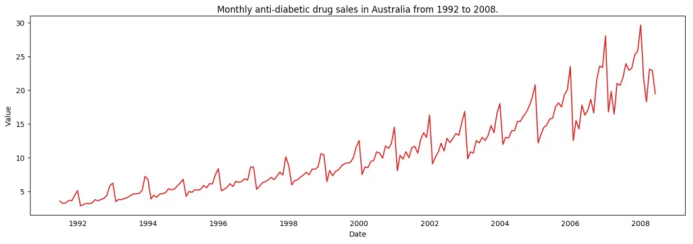时间序列可视化

因为所有的值都是正值，你可以在Y轴的两侧进行显示此值以强调增长。

# Import data
x = df['date'].values
y1 = df['value'].values

# Plot
fig, ax = plt.subplots(1, 1, figsize=(16,5), dpi= 120)
plt.fill_between(x, y1=y1, y2=-y1, alpha=0.5, linewidth=2, color='seagreen')
plt.ylim(-800, 800)
plt.title('Air Passengers (Two Side View)', fontsize=16)
plt.hlines(y=0, xmin=np.min(df.date), xmax=np.max(df.date), linewidth=.5)
plt.show()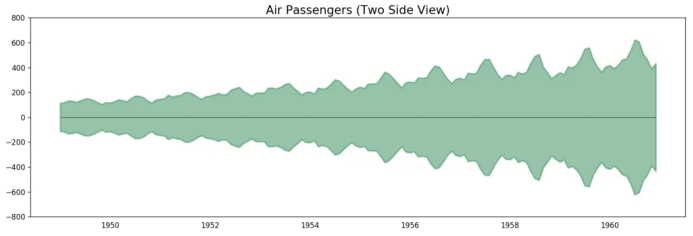航空乘客数据——两侧序列

因为这是一个月度时间序列，每年遵循特定的重复模式，你可以把每年作为一个单独的线画在同一张图上。这可以让你同时比较不同年份的模式。

4.1 时间序列的季节图

# Import Data
df.reset_index(inplace=True)

# Prepare data
df['year'] = [d.year for d in df.date]
df['month'] = [d.strftime('%b') for d in df.date]
years = df['year'].unique()

# Prep Colors
np.random.seed(100)
mycolors = np.random.choice(list(mpl.colors.XKCD_COLORS.keys()), len(years), replace=False)

# Draw Plot
plt.figure(figsize=(16,12), dpi= 80)
for i, y in enumerate(years):
if i > 0:
plt.plot('month', 'value', data=df.loc[df.year==y, :], color=mycolors[i], label=y)
plt.text(df.loc[df.year==y, :].shape-.9, df.loc[df.year==y, 'value'][-1:].values, y, fontsize=12, color=mycolors[i])

# Decoration
plt.gca().set(xlim=(-0.3, 11), ylim=(2, 30), ylabel='$Drug Sales$', xlabel='$Month$')
plt.yticks(fontsize=12, alpha=.7)
plt.title("Seasonal Plot of Drug Sales Time Series", fontsize=20)
plt.show()药品销售的季节图

每年二月会迎来药品销售的急速下降，而在三月会再度上升，接下来的4月又开始下降，以此类推。很明显，该模式在特定的某一年中重复，且年年如此。

然而，随着年份推移，药品销售整体增加。你可以很好地看到该趋势并且在年份箱线图当中看到它是怎样变化的。同样地，你也可以做一个月份箱线图来可视化月度分布情况。

4.2 月度（季节性）箱线图和年度（趋势）分布

你可以季节间隔将数据分组，并看看在给定的年份或月份当中值是如何分布的，以及随时间推移它们是如何比较的。

# Import Data
df.reset_index(inplace=True)

# Prepare data
df['year'] = [d.year for d in df.date]
df['month'] = [d.strftime('%b') for d in df.date]
years = df['year'].unique()

# Draw Plot
fig, axes = plt.subplots(1, 2, figsize=(20,7), dpi= 80)
sns.boxplot(x='year', y='value', data=df, ax=axes)
sns.boxplot(x='month', y='value', data=df.loc[~df.year.isin([1991, 2008]), :])

# Set Title
axes.set_title('Year-wise Box Plot\n(The Trend)', fontsize=18);
axes.set_title('Month-wise Box Plot\n(The Seasonality)', fontsize=18)
plt.show()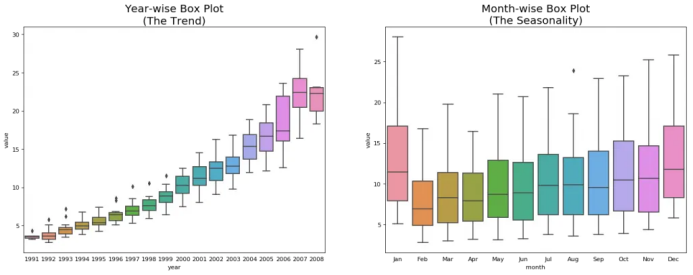年度和月度箱线图

箱线图将年度和月度分布变得很清晰。并且，在阅读箱线图当中，12月和1月明显有更高的药品销售量，可被归因于假期折扣季。

到目前为止，我们已经看到了识别模式的相似之处。现在怎样才能从通常模式当中找到离群值呢？

## 5. 时间序列的模式

任何时间序列都可以被分解为如下的部分：基线水平+趋势+季节性+误差

当在时间序列当中观测到增加或降低的斜率时，即可观测到相应的趋势。然而季节性只有在由于季节性因素导致不同的重复模式在规律性的间隔之间被观测到时才能发现。可能是由于当年的特定月份，特定月份的某一天、工作日或者甚至是当天某个时间。

然而，并不是所有时间序列必须有一个趋势和/或季节性。时间序列可能没有不同的趋势但是有一个季节性。反之亦然。

所以时间序列可以被看做是趋势、季节性和误差项的整合。

fig, axes = plt.subplots(1,3, figsize=(20,4), dpi=100)
pd.read_csv('https://raw.githubusercontent.com/selva86/datasets/master/guinearice.csv', parse_dates=['date'], index_col='date').plot(title='Trend Only', legend=False, ax=axes)

pd.read_csv('https://raw.githubusercontent.com/selva86/datasets/master/sunspotarea.csv', parse_dates=['date'], index_col='date').plot(title='Seasonality Only', legend=False, ax=axes)

pd.read_csv('https://raw.githubusercontent.com/selva86/datasets/master/AirPassengers.csv', parse_dates=['date'], index_col='date').plot(title='Trend and Seasonality', legend=False, ax=axes)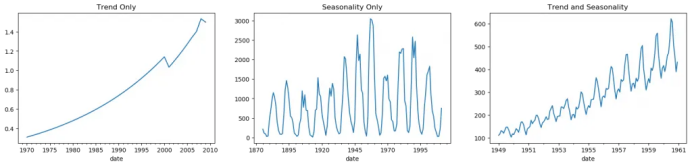时间序列中的模式

另一个需要考虑的方面是循环的行为。当序列当中上升和下降模式并不在固定的日历间隔出现时，就会出现循环的行为。需注意不要混淆循环的效应和季节的效应。

所以，怎样区分循环的和季节性的模式呢？

如果模式不是基于固定的日历频率，那它就是循环的。因为，循环效应不像季节性那样受到商业和其他社会经济因素的影响。

## 6. 时间序列的加法和乘法

基于趋势和季节性的本质，时间序列以加法或乘法的形式建模，其中序列里的每个观测值可被表达为成分的和或者积：

加法时间序列：值=基线水平+趋势+季节性+误差

乘法时间序列：值=基线水平*趋势*季节性*误差

## 7. 怎样分解时间序列的成分？

你可以通过将序列作基线水平，趋势，季节性指数和残差的加法或乘法组合来实现一个经典的时间序列分解。

statsmodels包里的seasonal_decompose使用起来非常方便。

from statsmodels.tsa.seasonal import seasonal_decompose
from dateutil.parser import parse

# Import Data

# Multiplicative Decomposition
result_mul = seasonal_decompose(df['value'], model='multiplicative', extrapolate_trend='freq')

# Plot
plt.rcParams.update({'figure.figsize': (10,10)})
result_mul.plot().suptitle('Multiplicative Decompose', fontsize=22)
plt.show()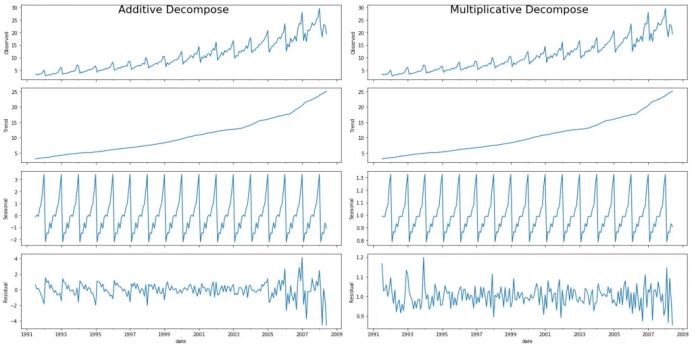加法和乘法分解

在序列开始时，设置extrapolate_trend='freq' 来注意趋势和残差中缺失的任何值。

如果你仔细看加法分解当中的残差，它有一些遗留模式。乘法分解看起来非常随意，这很好。所以理想状况下，乘法分解应该在这种特定的序列当中优先选择。

趋势，季节性和残差成分的数值输出被存储在result_mul 当中。让我们提取它们并导入数据框中。

# Extract the Components ----
# Actual Values = Product of (Seasonal * Trend * Resid)
df_reconstructed = pd.concat([result_mul.seasonal, result_mul.trend, result_mul.resid, result_mul.observed], axis=1)
df_reconstructed.columns = ['seas', 'trend', 'resid', 'actual_values']


如果你检查一下seas, trend 和 resid列的乘积，应该确实等于actual_values。

## 8. 平稳和非平稳时间序列

平稳性是时间序列的属性之一。平稳序列的值不是时间的函数。

也就是说，这种序列的统计属性例如均值，方差和自相关是随时间不变的常数。序列的自相关只是与前置值的相关，之后会详细介绍。

平稳时间序列也没有季节效应。

所以如何识别一个序列是否平稳呢？让我们通过实例来展示一下：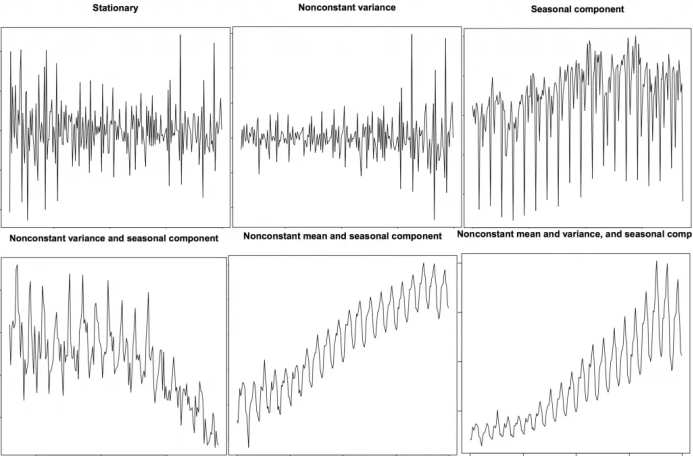平稳和非平稳时间序列

上图来自R语言的 TSTutorial。

所以为什么平稳序列是重要的呢？为什么我要提到它？

我将展开讲一下，但是要理解它只是有可能通过使用特定的转换方法实现任何时间序列的平稳化。大多数统计预测方法都用于平稳时间序列。预测的第一步通常是做一些转换将非平稳数据转化为平稳数据。

## 9. 如何获取平稳的时间序列？

你可以通过以下步骤实现序列的平稳化：

1. 差分序列（一次或多次）；

2. 对序列值进行log转换；

3. 对序列值去n次根式值；

4. 结合上述方法。

实现数据平稳化最常见也最方便的方法是对序列进行差分至少一次，直到它变得差不多平稳为止。

9.1 所以什么是差分？

如果Y_t是t时刻的Y值，那么第一次差分Y = Yt – Yt-1。在简化的格式当中，差分序列就是从当前值中减去下一个值。

如果第一次差分不能使数据平稳，你可以第二次差分，以此类推。

例如，考虑如下序列: [1, 5, 2, 12, 20]

一次差分: [5-1, 2-5, 12-2, 20-12] = [4, -3, 10, 8]

二次差分: [-3-4, -10-3, 8-10] = [-7, -13, -2]

## 9.2 为什么要在预测之前将非平稳数据平稳化？

预测平稳序列相对容易，预测也相对更可靠。

一个重要的原因是自回归预测模型必须是利用序列自身的滞后量作为预测变量的线性回归模型。

我们知道线性回归在预测变量（X变量）与其他变量不相关时效果最佳。所以序列平稳化也因为移除所有持续的自相关而解决了这个问题，因此使得模型中的预测变量（序列的滞后值）几乎独立。

现在我们已经建立了序列平稳化非常重要的概念，那怎样检验给定序列是否平稳化呢？

## 10. 怎样检验平稳性?

序列的平稳性可以通过之前我们提到的序列图看出来。

另外一种方法是将序列分成2或多个连续的部分，计算概要统计量例如均值，方差和自相关。如果统计量显著差异，序列可能不是平稳的。

尽管如此，你需要一个方法来从量化的角度判断一个给定序列是否平稳。可以通过‘Unit Root Tests单位根检验’来实现。这里有多种变式，但这些检验都是用来检测时间序列是否非平稳并且拥有一个单位根。

有多种单位根检验的具体应用：

2. 科维亚特夫斯基-菲利普斯-施密特-辛-KPSS检验（趋势平稳性）；

3. 菲利普斯 佩龙检验（PP Test）。

from statsmodels.tsa.stattools import adfuller, kpss

print(f'p-value: {result}')
for key, value in result.items():
print('Critial Values:')
print(f'   {key}, {value}')

# KPSS Test
result = kpss(df.value.values, regression='c')
print('\nKPSS Statistic: %f' % result)
print('p-value: %f' % result)
for key, value in result.items():
print('Critial Values:')
print(f'   {key}, {value}')
p-value: 1.0
Critial Values:
1%, -3.465620397124192
Critial Values:
5%, -2.8770397560752436
Critial Values:
10%, -2.5750324547306476

KPSS Statistic: 1.313675
p-value: 0.010000
Critial Values:
10%, 0.347
Critial Values:
5%, 0.463
Critial Values:
2.5%, 0.574
Critial Values:
1%, 0.739


## 11. 白噪音和平稳序列的差异是什么？

如平稳序列，白噪音也不是时间的函数，它的均值和方差并不随时间变化。但是它与平稳序列的差异在于，白噪音完全随机，均值为0。

无论怎样，在白噪音当中是没有特定模式的。如果你将FM广播的声音信号作为时间序列，你在频道之间的频段听到的空白声就是白噪音。

从数学上来看，均值为0的完全随机的数字序列是白噪音。

randvals = np.random.randn(1000)
pd.Series(randvals).plot(title='Random White Noise', color='k')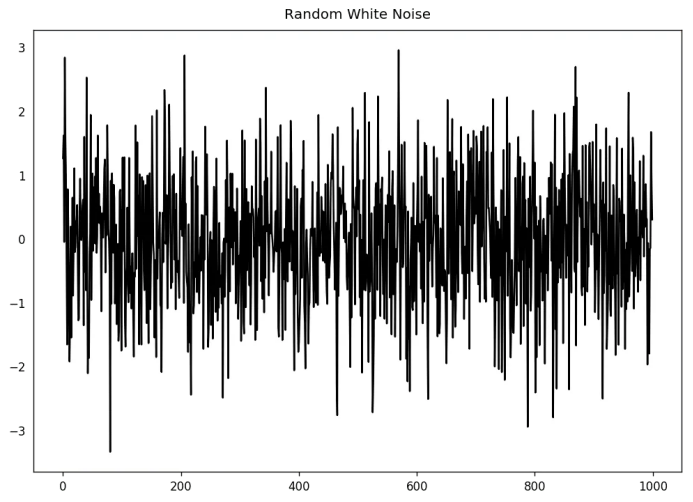随机白噪音

## 12. 怎样将时间序列去趋势化？

对时间序列去趋势就是从时间序列当中移除趋势成分。但是如何提取趋势呢？有以下几个方法。

1. 从时间序列当中减去最优拟合线。最佳拟合线可从以时间步长为预测变量获得的线性回归模型当中获得。对更复杂的模型，你可以使用模型中的二次项（x^2）；

2. 从我们之前提过的时间序列分解当中减掉趋势成分；

3. 减去均值；

4. 应用像Baxter-King过滤器(statsmodels.tsa.filters.bkfilter)或者Hodrick-Prescott 过滤器 (statsmodels.tsa.filters.hpfilter)来去除移动的平均趋势线或者循环成分。

让我们来用一下前两种方法。

# Using scipy: Subtract the line of best fit
from scipy import signal
detrended = signal.detrend(df.value.values)
plt.plot(detrended)
plt.title('Drug Sales detrended by subtracting the least squares fit', fontsize=16)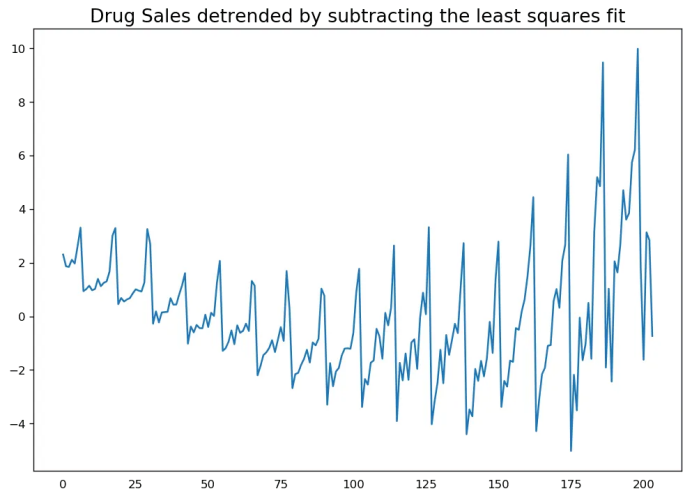通过减去最小二乘拟合来对时间序列去趋势化

# Using statmodels: Subtracting the Trend Component.
from statsmodels.tsa.seasonal import seasonal_decompose
result_mul = seasonal_decompose(df['value'], model='multiplicative', extrapolate_trend='freq')
detrended = df.value.values - result_mul.trend
plt.plot(detrended)
plt.title('Drug Sales detrended by subtracting the trend component', fontsize=16)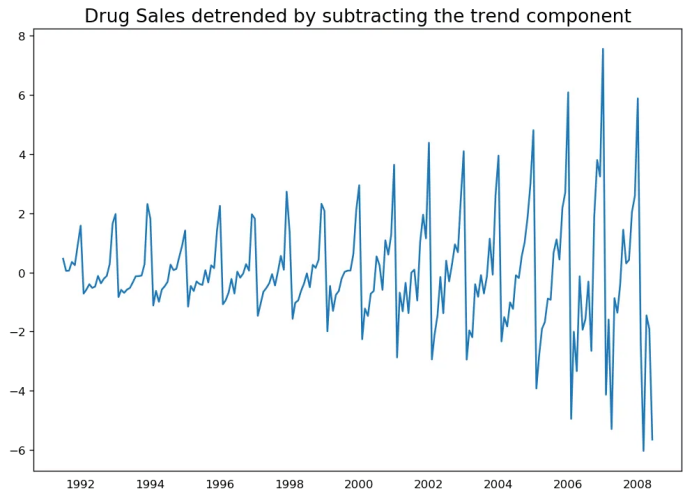通过减去趋势成分来去趋势化

## 13. 怎样对时间序列去季节化?

这里有多种方法对时间序列去季节化。以下就有几个：

1. 取一个以长度为季节窗口的移动平均线。这将在这个过程中使序列变得平滑；

2. 序列季节性差分（从当前值当中减去前一季节的值）；

3. 将序列值除以从STL分解当中获得的季节性指数。

如果除以季节性指数后仍没办法得到良好的结果，再试一下序列对数转换然后再做。你之后可以通过去指数恢复到原始尺度。

# Subtracting the Trend Component.

# Time Series Decomposition
result_mul = seasonal_decompose(df['value'], model='multiplicative', extrapolate_trend='freq')

# Deseasonalize
deseasonalized = df.value.values / result_mul.seasonal

# Plot
plt.plot(deseasonalized)
plt.title('Drug Sales Deseasonalized', fontsize=16)
plt.plot()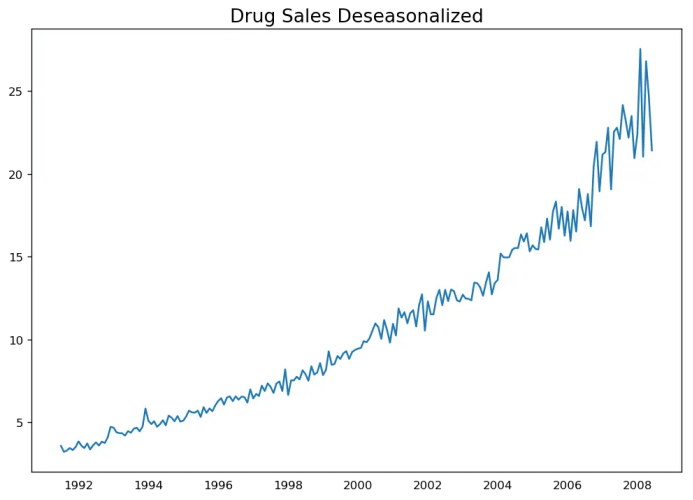时间序列去季节化

## 14. 怎样检验时间序列的季节性？

常见的方法是绘制序列并在固定的时间间隔内检查可重复的模式。所以，季节性的类型由钟表或日历决定：

1. 一天的每个小时；

2. 一月的每天；

3. 每周；

4. 每月；

5. 每年。

然而，如果你想要一个更权威的季节性检验，使用自回归函数（ACF）图。更多关于自回归的信息将在下一部分介绍。但是当强季节性模式出现时，ACF图通常揭示了在季节窗的倍数处明显的重复峰值。

例如，药品销售时间序列是每年都有重复模式的一个月度序列。所以，你可以看到第12,24和36条线等的峰值。

我必须警告你在现实世界的数据集当中，这样强的模式很难见到，并且有可能被各种噪音所扭曲，所以你需要一双仔细的眼睛来捕获这些模式。

from pandas.plotting import autocorrelation_plot

# Draw Plot
plt.rcParams.update({'figure.figsize':(9,5), 'figure.dpi':120})
autocorrelation_plot(df.value.tolist())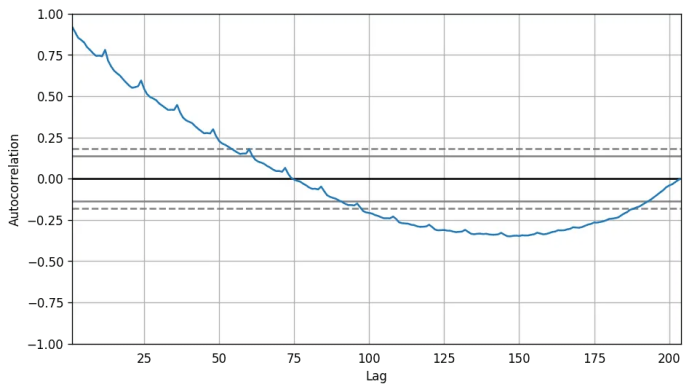自相关图

除此之外，如果你想做统计检验，CHT检验可以检验季节性差异是否对序列平稳化有必要。

## 15. 如何处理时间序列当中的缺失值？

有时，你的时间序列会有缺失日期/时间。那意味着，数据没有被捕获或者在那段时间内不可用。那些天的测量值有可能为0，你可以把那些时间段填充0。

其次，当处理时间序列时，你通常不应该用序列均值来替代缺失值，尤其是序列非平稳的时候，一个快捷粗略的处理方法来说你应该做的是向前填充之前的值。

然而，依赖于序列的本质，你想要在得出结论之前尝试多种方法。有效的缺失值处理方法有：

• 向后填充；

• 线性内插；

• 二次内插；

• 最邻近平均值；

• 对应季节的平均值。

为了衡量缺失值的表现，我在时间序列当中手动引入缺失值，使用上述方法处理并衡量处理值和真实值之间的均方误差。

# # Generate dataset
from scipy.interpolate import interp1d
from sklearn.metrics import mean_squared_error

fig, axes = plt.subplots(7, 1, sharex=True, figsize=(10, 12))
plt.rcParams.update({'xtick.bottom' : False})

## 1. Actual -------------------------------
df_orig.plot(title='Actual', ax=axes, label='Actual', color='red', style=".-")
df.plot(title='Actual', ax=axes, label='Actual', color='green', style=".-")
axes.legend(["Missing Data", "Available Data"])

## 2. Forward Fill --------------------------
df_ffill = df.ffill()
error = np.round(mean_squared_error(df_orig['value'], df_ffill['value']), 2)
df_ffill['value'].plot(title='Forward Fill (MSE: ' + str(error) +")", ax=axes, label='Forward Fill', style=".-")

## 3. Backward Fill -------------------------
df_bfill = df.bfill()
error = np.round(mean_squared_error(df_orig['value'], df_bfill['value']), 2)
df_bfill['value'].plot(title="Backward Fill (MSE: " + str(error) +")", ax=axes, label='Back Fill', color='firebrick', style=".-")

## 4. Linear Interpolation ------------------
df['rownum'] = np.arange(df.shape)
df_nona = df.dropna(subset = ['value'])
f = interp1d(df_nona['rownum'], df_nona['value'])
df['linear_fill'] = f(df['rownum'])
error = np.round(mean_squared_error(df_orig['value'], df['linear_fill']), 2)
df['linear_fill'].plot(title="Linear Fill (MSE: " + str(error) +")", ax=axes, label='Cubic Fill', color='brown', style=".-")

## 5. Cubic Interpolation --------------------
f2 = interp1d(df_nona['rownum'], df_nona['value'], kind='cubic')
df['cubic_fill'] = f2(df['rownum'])
error = np.round(mean_squared_error(df_orig['value'], df['cubic_fill']), 2)
df['cubic_fill'].plot(title="Cubic Fill (MSE: " + str(error) +")", ax=axes, label='Cubic Fill', color='red', style=".-")

# Interpolation References:
# https://docs.scipy.org/doc/scipy/reference/tutorial/interpolate.html
# https://docs.scipy.org/doc/scipy/reference/interpolate.html

## 6. Mean of 'n' Nearest Past Neighbors ------
def knn_mean(ts, n):
out = np.copy(ts)
for i, val in enumerate(ts):
if np.isnan(val):
n_by_2 = np.ceil(n/2)
lower = np.max([0, int(i-n_by_2)])
upper = np.min([len(ts)+1, int(i+n_by_2)])
ts_near = np.concatenate([ts[lower:i], ts[i:upper]])
out[i] = np.nanmean(ts_near)
return out

df['knn_mean'] = knn_mean(df.value.values, 8)
error = np.round(mean_squared_error(df_orig['value'], df['knn_mean']), 2)
df['knn_mean'].plot(title="KNN Mean (MSE: " + str(error) +")", ax=axes, label='KNN Mean', color='tomato', alpha=0.5, style=".-")

## 7. Seasonal Mean ----------------------------
def seasonal_mean(ts, n, lr=0.7):
"""
Compute the mean of corresponding seasonal periods
ts: 1D array-like of the time series
n: Seasonal window length of the time series
"""
out = np.copy(ts)
for i, val in enumerate(ts):
if np.isnan(val):
ts_seas = ts[i-1::-n]  # previous seasons only
if np.isnan(np.nanmean(ts_seas)):
ts_seas = np.concatenate([ts[i-1::-n], ts[i::n]])  # previous and forward
out[i] = np.nanmean(ts_seas) * lr
return out

df['seasonal_mean'] = seasonal_mean(df.value, n=12, lr=1.25)
error = np.round(mean_squared_error(df_orig['value'], df['seasonal_mean']), 2)
df['seasonal_mean'].plot(title="Seasonal Mean (MSE: " + str(error) +")", ax=axes, label='Seasonal Mean', color='blue', alpha=0.5, style=".-")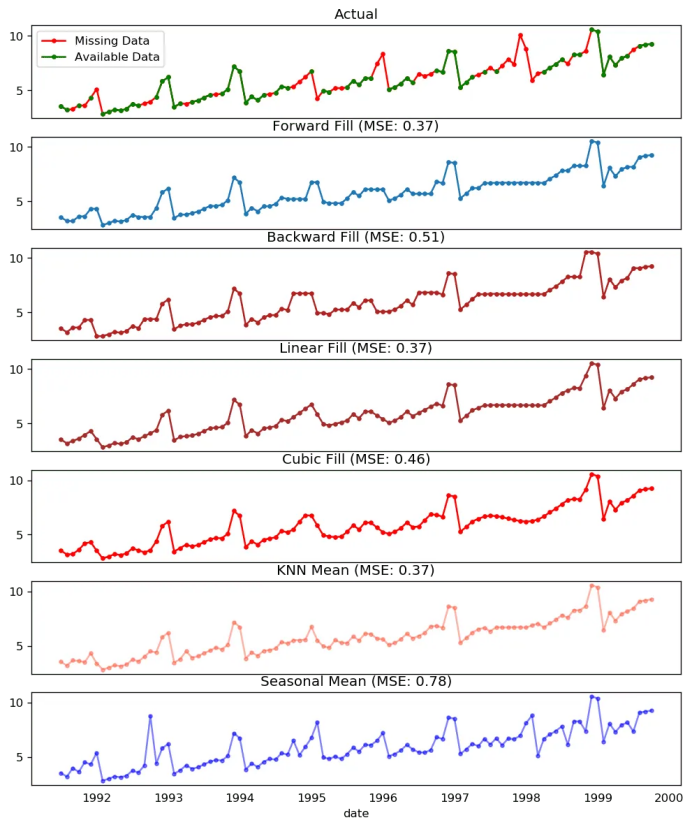缺失值处理

你也可以根据你想实现的精确程度考虑接下来的方法。

1. 如果你有解释变量，可以使用像随机森林或k-邻近算法的预测模型来预测它。

2. 如果你有足够多的过去观测值，可以预测缺失值。

3. 如果你有足够的未来观测值，回测缺失值。

4. 从之前的周期预测相对应的部分。

## 16. 什么是自相关和偏自相关函数？

自相关是序列和自己滞后量的简单相关。如果序列显著自相关，均值和序列之前的值（滞后量）可能对预测当前值有帮助。

偏自相关也会传递相似的信息但是它传递的是序列和它滞后量的纯粹相关，排除了其他中间滞后量对相关的贡献。

from statsmodels.tsa.stattools import acf, pacf
from statsmodels.graphics.tsaplots import plot_acf, plot_pacf

# Calculate ACF and PACF upto 50 lags
# acf_50 = acf(df.value, nlags=50)
# pacf_50 = pacf(df.value, nlags=50)

# Draw Plot
fig, axes = plt.subplots(1,2,figsize=(16,3), dpi= 100)
plot_acf(df.value.tolist(), lags=50, ax=axes)
plot_pacf(df.value.tolist(), lags=50, ax=axes)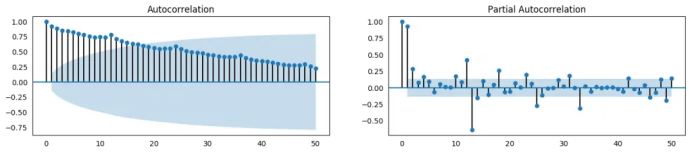自相关函数 和 偏自相关函数

## 17. 怎样计算偏自相关函数?

怎样计算偏自相关呢?

序列滞后量（k）的偏自相关是Y的自回归方程中滞后量的系数。Y的自回归方程就是Y及其滞后量作为预测项的线性回归。

For Example, if Y_t is the current series and Y_t-1 is the lag 1 of Y, then the partial autocorrelation of lag 3 (Y_t-3) is the coefficient $\alpha_3$ of Y_t-3 in the following equation:

例如，如果Y_t是当前的序列，Y_t-1是Y的滞后量1，那么滞后量3（Y_t-3）的偏自相关就是下面方程中Y_t-3的系数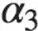: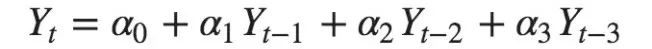自回归方程

## 18. 滞后图

滞后图是一个时间序列对其自身滞后量的散点图。它通常用于检查自相关。如果序列中存在如下所示的任何模式，则该序列是自相关的。如果没有这样的模式，这个序列很可能是随机的白噪声。

在下面太阳黑子面积时间序列的例子当中，随着n_lag增加，图越来越分散。

from pandas.plotting import lag_plot

# Import

# Plot
fig, axes = plt.subplots(1, 4, figsize=(10,3), sharex=True, sharey=True, dpi=100)
for i, ax in enumerate(axes.flatten()[:4]):
lag_plot(ss.value, lag=i+1, ax=ax, c='firebrick')
ax.set_title('Lag ' + str(i+1))

fig.suptitle('Lag Plots of Sun Spots Area \n(Points get wide and scattered with increasing lag -> lesser correlation)\n', y=1.15)

fig, axes = plt.subplots(1, 4, figsize=(10,3), sharex=True, sharey=True, dpi=100)
for i, ax in enumerate(axes.flatten()[:4]):
lag_plot(a10.value, lag=i+1, ax=ax, c='firebrick')
ax.set_title('Lag ' + str(i+1))

fig.suptitle('Lag Plots of Drug Sales', y=1.05)
plt.show()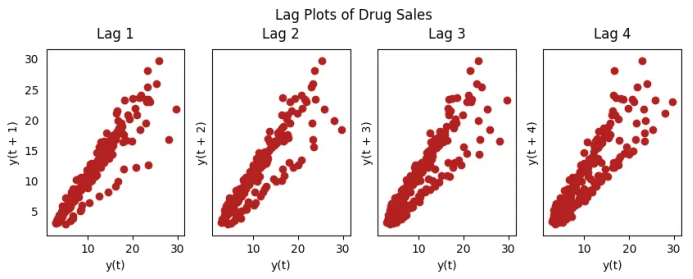药品销售的滞后图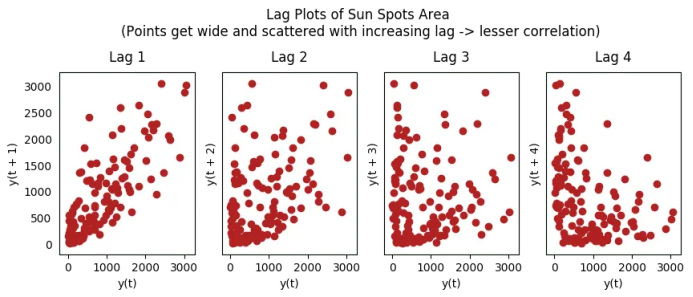太阳黑子的滞后图

## 19. 怎样估计时间序列的预测能力?

时间序列越有规律性和重复性的模式，越容易被预测。“近似熵”可用于量化时间序列波动的规律性和不可预测性。

近似熵越高，预测越难。另一个更好的选项是“样本熵”。

样本熵类似与近似熵，但是在估计小时间序列的复杂性上结果更一致。例如，较少样本点的随机时间序列 “近似熵”可能比一个更规律的时间序列更低，然而更长的时间序列可能会有一个更高的“近似熵”。

样本熵可以很好地处理这个问题。请看如下演示：

# https://en.wikipedia.org/wiki/Approximate_entropy
rand_small = np.random.randint(0, 100, size=36)
rand_big = np.random.randint(0, 100, size=136)

def ApEn(U, m, r):
"""Compute Aproximate entropy"""
def _maxdist(x_i, x_j):
return max([abs(ua - va) for ua, va in zip(x_i, x_j)])

def _phi(m):
x = [[U[j] for j in range(i, i + m - 1 + 1)] for i in range(N - m + 1)]
C = [len([1 for x_j in x if _maxdist(x_i, x_j) <= r]) / (N - m + 1.0) for x_i in x]
return (N - m + 1.0)**(-1) * sum(np.log(C))

N = len(U)
return abs(_phi(m+1) - _phi(m))

print(ApEn(ss.value, m=2, r=0.2*np.std(ss.value)))     # 0.651
print(ApEn(a10.value, m=2, r=0.2*np.std(a10.value)))   # 0.537
print(ApEn(rand_small, m=2, r=0.2*np.std(rand_small))) # 0.143
print(ApEn(rand_big, m=2, r=0.2*np.std(rand_big)))     # 0.716
0.6514704970333534
0.5374775224973489
0.0898376940798844
0.7369242960384561
# https://en.wikipedia.org/wiki/Sample_entropy
def SampEn(U, m, r):
"""Compute Sample entropy"""
def _maxdist(x_i, x_j):
return max([abs(ua - va) for ua, va in zip(x_i, x_j)])

def _phi(m):
x = [[U[j] for j in range(i, i + m - 1 + 1)] for i in range(N - m + 1)]
C = [len([1 for j in range(len(x)) if i != j and _maxdist(x[i], x[j]) <= r]) for i in range(len(x))]
return sum(C)

N = len(U)
return -np.log(_phi(m+1) / _phi(m))

print(SampEn(ss.value, m=2, r=0.2*np.std(ss.value)))      # 0.78
print(SampEn(a10.value, m=2, r=0.2*np.std(a10.value)))    # 0.41
print(SampEn(rand_small, m=2, r=0.2*np.std(rand_small)))  # 1.79
print(SampEn(rand_big, m=2, r=0.2*np.std(rand_big)))      # 2.42
0.7853311366380039
0.41887013457621214
inf
2.181224235989778

del sys.path


## 20. 为何要以及怎样对时间序列进行平滑处理?

时间序列平滑处理可能在以下场景有用：

• 在信号当中减小噪声的影响从而得到一个经过噪声滤波的序列近似。

• 平滑版的序列可用于解释原始序列本身的特征。

• 趋势更好地可视化。

怎样对序列平滑处理？让我们讨论一下以下方法：

1. 使用移动平均；

2. 做LOESS光滑（局部回归）；

3. 做LOWESS光滑（局部加权回归）。

移动均值就是定义宽度的滚动窗口的均值。但是你必须明智地选择窗口宽度，因为大范围窗口可能会造成序列过度平滑。例如，窗口大小等于季节持续时间时（例如：12为月度序列），将有效地抵消季节效应。

LOESS，局部回归的简写，适应于每个点邻近的多元回归。可通过statsmodels包使用，你可以使用frac参数确定被纳入拟合回归模型的邻近数据点的百分比来控制平滑度。

下载数据集: Elecequip.csv

from statsmodels.nonparametric.smoothers_lowess import lowess

# Import

# 1. Moving Average
df_ma = df_orig.value.rolling(3, center=True, closed='both').mean()

# 2. Loess Smoothing (5% and 15%)
df_loess_5 = pd.DataFrame(lowess(df_orig.value, np.arange(len(df_orig.value)), frac=0.05)[:, 1], index=df_orig.index, columns=['value'])
df_loess_15 = pd.DataFrame(lowess(df_orig.value, np.arange(len(df_orig.value)), frac=0.15)[:, 1], index=df_orig.index, columns=['value'])

# Plot
fig, axes = plt.subplots(4,1, figsize=(7, 7), sharex=True, dpi=120)
df_orig['value'].plot(ax=axes, color='k', title='Original Series')
df_loess_5['value'].plot(ax=axes, title='Loess Smoothed 5%')
df_loess_15['value'].plot(ax=axes, title='Loess Smoothed 15%')
df_ma.plot(ax=axes, title='Moving Average (3)')
fig.suptitle('How to Smoothen a Time Series', y=0.95, fontsize=14)
plt.show()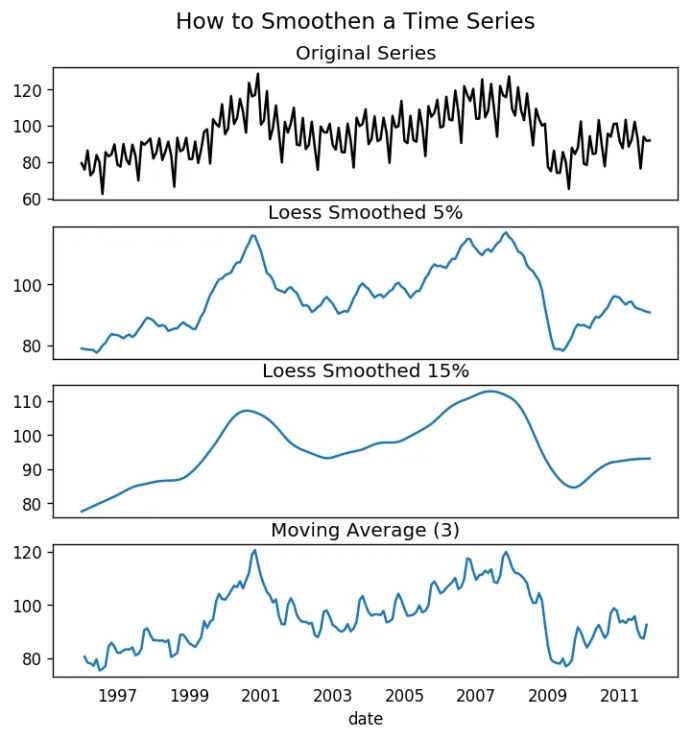平滑时间序列

## 21. 如何使用Granger因果检验得知是否一个时间序列有助于预测另一个序列？

Granger因果检验被用于检验是否一个时间序列可以预测另一个序列。Granger因果检验是如何工作的？

它基于如果X引起Y的变化，Y基于之前的Y值和之前的X值的预测效果要优于仅基于之前的Y值的预测效果。

所以需要了解Granger因果检验不能应用于Y的滞后量引起Y自身的变化的情况，而通常仅用于外源变量（不是Y的滞后量）。

它在statsmodel包中得到了很好的实现。它采纳2列数据的二维数组作为主要参数，被预测值是第一列，而预测变量（X）在第二列。

零假设检验：第二列的序列不能Granger预测第一列数据。如果p值小于显著性水平（0.05），你可以拒绝零假设并得出结论：X的滞后量确实有用。

第二个参数maxlag决定有多少Y的滞后量应该纳入检验当中。

from statsmodels.tsa.stattools import grangercausalitytests
df['month'] = df.date.dt.month
grangercausalitytests(df[['value', 'month']], maxlag=2)
Granger Causality
number of lags (no zero) 1
ssr based F test:         F=54.7797 , p=0.0000  , df_denom=200, df_num=1
ssr based chi2 test:   chi2=55.6014 , p=0.0000  , df=1
likelihood ratio test: chi2=49.1426 , p=0.0000  , df=1
parameter F test:         F=54.7797 , p=0.0000  , df_denom=200, df_num=1

Granger Causality
number of lags (no zero) 2
ssr based F test:         F=162.6989, p=0.0000  , df_denom=197, df_num=2
ssr based chi2 test:   chi2=333.6567, p=0.0000  , df=2
likelihood ratio test: chi2=196.9956, p=0.0000  , df=2
parameter F test:         F=162.6989, p=0.0000  , df_denom=197, df_num=2


在上述例子当中，实际上所有检验的p值只能无限接近于0。所以“月份”实际上可以用于预测航空乘客的数量。

## 22. 下一步是什么？

这就是我们现在要说的。我们从非常基础的内容开始，理解了时间序列不同特征。一旦分析完成之后，接下来的一步是预测。

原文标题：

Time Series Analysis in Python – A Comprehensive Guide with Examples

原文链接：

https://www.machinelearningplus.com/time-series/time-series-analysis-python/

编辑：黄继彦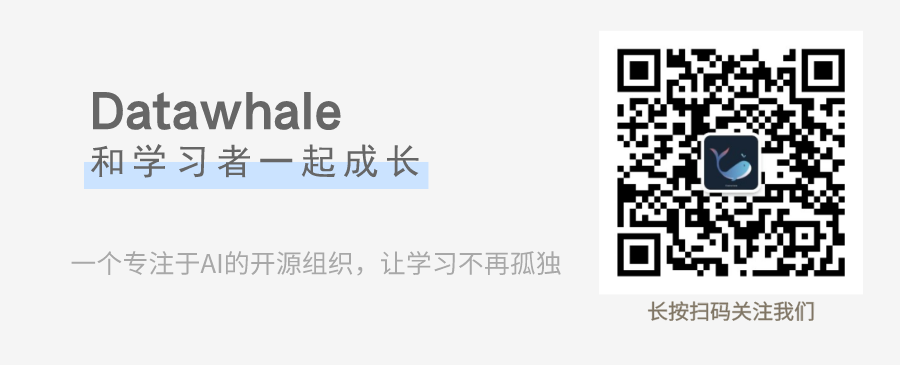干货学习，三连

展开全文python 机器学习 深度学习
• 时间序列是将统一统计值按照时间发生的先后顺序来进行排列，时间序列分析的主要目的是根据已有数据对未来进行预测。 一稳定的时间序列中常常包含两部分，那么就是：有规律的时间序列+噪声。所以，在以下的方法中...

## 一、时间序列的定义

时间序列是将统一统计值按照时间发生的先后顺序来进行排列，时间序列分析的主要目的是根据已有数据对未来进行预测。

一个稳定的时间序列中常常包含两个部分，那么就是：有规律的时间序列+噪声。所以，在以下的方法中，主要的目的就是去过滤噪声值，让我们的时间序列更加的有分析意义。

## 二、时间序列的预处理

1、平稳性检验：

拿到一个时间序列之后，我们首先要对其稳定性进行判断，只有非白噪声的稳定性时间序列才有分析的意义以及预测未来数据的价值。

所谓平稳，是指统计值在一个常数上下波动并且波动范围是有界限的。如果有明显的趋势或者周期性，那么就是不稳定的。一般判断有三种方法：

在R语言中，DF检测是一种检测稳定性的方法，如果得出的P值小于临界值，则认为是数列是稳定的。

2、白噪声检验

白噪声序列，又称为纯随机性序列，序列的各个值之间没有任何的相关关系，序列在进行无序的随机波动，可以终止对该序列的分析，因为从白噪声序列中是提取不到任何有价值的信息的。

3、平稳时间序列的参数特点

均值和方差为常数，并且具有与时间无关的自协方差。

## 三、时间序列建模步骤

• 拿到被分析的时间序列数据集。
• 对数据绘图，观测其平稳性。若为非平稳时间序列要先进行d阶差分运算后化为平稳时间序列，此处的d即为ARIMA(p,d,q)模型中的d；若为平稳序列，则用ARMA(p,q)模型。所以ARIMA(p,d,q) 模型区别于ARMA(p,q)之处就在于前者的自回归部分的特征多项式含有d个单位根。
• 对得到的平稳时间序列分别求得其自相关系数ACF 和偏自相关系数PACF，通过对自相关图和偏自相关图的分析，得到最佳的阶层 p 和阶数 q。由以上得到的d、q、p ，得到ARIMA模型。
• 模型诊断。进行诊断分析，以证实所得模型确实与所观察到的数据特征相符。若不相符，重新回到第（3）步。

## 四、自回归综合移动平均模型ARIMA

首先我们先了解AR（自回归模型）和MA（移动平均模型）

AR(自回归模型):当存在xt = f1 xt-1 + f2 xt-2 + …+fp xt-p+ut .时，其中ut是白噪声过程，则这个线性过程成为p阶自回归过程。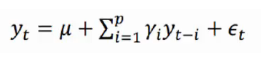一般我们常用的即为1阶以及2阶自回归即：xt = f1 xt-1 + ut，xt = f1 xt-1 + f2 xt-2 + ut。

自回归模型平稳必要非充分条件为所有系数相加<1

MA（移动平均模型）:移动平均即将时间序列中的若干项进行算术平均得到的一系列平均数，若平均的数据项数为k，则成为k期的平均移动。相应的，还有加权平均移动法，即将特定期数的值进行加权计算平均值。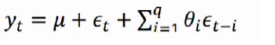ARMA(自回归移动平均模型)：
自回归滑动平均模型（ARMA）
如果时间序列Yt满足：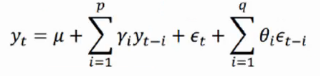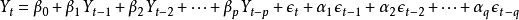则称时间序列为服从(p,q)阶自回归滑动平均混合模型。或者记为φ(B)
（摘自百度百科，MA的体现形式有所出入，请仔细体会）

ARIMA(自回归差分移动平均模型):
有了以上基础之后，我们便很容易理解ARIMA模型了。

前边我们提到，非稳定性模型可以通过差分的方式使之平稳化，而ARIMA即在参数中比ARMA多了一个差分过程，如果ARMA的公式为ARMA(P,Q)，那么ARIMA即为ARIMA(P,D,Q)这里的d即为差分的意思，代表是几阶差分。

## 五、指数平滑法

基本原理：指数平滑法是移动平均法中的一种，其特点在于给过去的观测值不一样的权重，即较近期观测值的权数比较远期观测值的权数要大。根据平滑次数不同，指数平滑法分为一次指数平滑法、二次指数平滑法和三次指数平滑法等。但它们的基本思想都是：预测值是以前观测值的加权和，且对不同的数据给予不同的权数，新数据给予较大的权数，旧数据给予较小的权数。
方法应用：指数平滑法是生产预测中常用的一种方法。也用于中短期经济发展趋势预测，所有预测方法中，指数平滑是用得最多的一种。
指数平滑法的基本公式：St=a*yt+(1-a)*St-1 式中，
St–时间t的平滑值；
yt–时间t的实际值；
St-1–时间t-1的平滑值；
a–平滑常数，其取值范围为[0,1]

据平滑次数不同，指数平滑法分为：一次指数平滑法、二次指数平滑和三次指数平滑法等。

## 六、R语言中实现案例

#时间序列相关案例

#ts方法构建时间序列介绍
a<-ts(1:30,frequency = 12,start = c(2011,3))
print(a)
# > print(a)
# Jan Feb Mar Apr May Jun Jul Aug Sep Oct Nov Dec
# 2011           1   2   3   4   5   6   7   8   9  10
# 2012  11  12  13  14  15  16  17  18  19  20  21  22
# 2013  23  24  25  26  27  28  29  30
str(a)
attributes(a)

#在ts函数的参数中，1：30代以1-30为基础建立30个时间序列值，frequence=7代表以周循环，=12代表以月循环，=4代表以季度循环
#start 代表起始的日期


#将时间序列拆分成趋势成分、季节成分、随机成分
#所谓的趋势成分是指长时间间隔的大体运动方向，季节成分是指季节性的变化，随机成分是指重复但非周期的波动，最终是随机成分
#R自带的AirPassengers中很好演示了时间序列
plot(AirPassengers)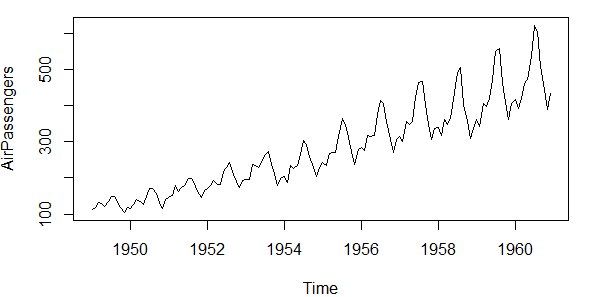install.packages("tseries")
install.packages("forecast")
library(tseries)
library(forecast)
#同样可以使用tsdisplay函数显示ACF和PACF图，用来确定arima函数的参数
tsdisplay(AirPassengers)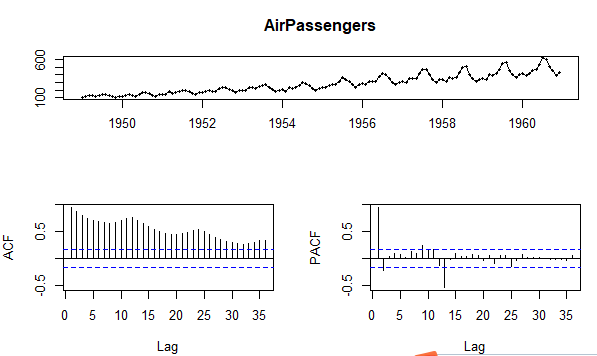#使用decompose()函数将其拆分为不同成分
dc<-decompose(AirPassengers)

#打印出四种趋势图
plot(dc)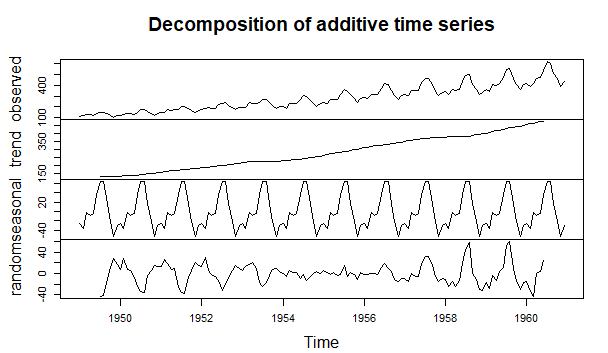#打印出关于季节性趋势的图表
season<-dc$figure plot(season,type = "b",xaxt="n",xlab = "")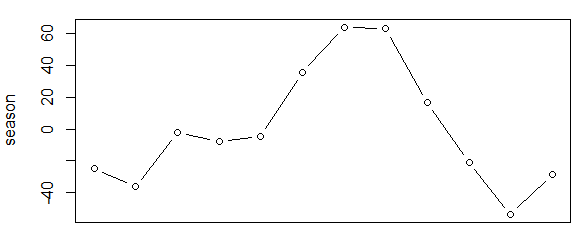#构建arima模型，我们首先使用forcast包里的auto.arima进行参数估计，然后再使用arima a<-auto.arima(AirPassengers) print(a) # > print(a) # Series: AirPassengers # ARIMA(2,1,1)(0,1,0) # # Coefficients: # ar1 ar2 ma1 # 0.5960 0.2143 -0.9819 # s.e. 0.0888 0.0880 0.0292 # # sigma^2 estimated as 132.3: log likelihood=-504.92 # AIC=1017.85 AICc=1018.17 BIC=1029.35  fit<-arima(AirPassengers,order = c(2,1,1),seasonal = list(order=c(0,1,0),period=12)) print(fit) fore=predict(fit,n.head=24) U<-fore$pred+2*fore$se L<-fore$pred-2*fore$se ts.plot(AirPassengers,fore$pred,U,L,col=c(1,2,4,4),lty=c(1,1,2,2))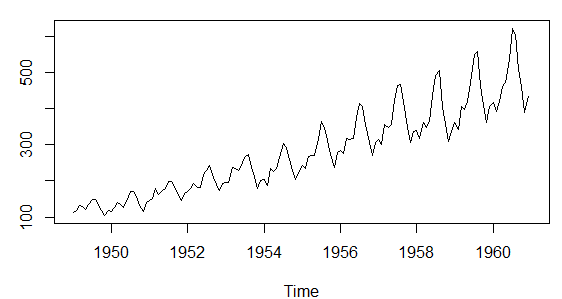## 参考书目：

A Complete Tutorial on Time Series Modeling in R

《时间序列预测实践教程》-清华大学出版社

《时间序列分析28讲视频》-中国科技大学

展开全文r语言
• 时间序列分析及应用（R语言）（原书第2版）》的另一特点是包含很有用的附录．例如，回顾了有关期望、方差、协方差、相关系数等概念．筒述了条件期望的性质以及最小均方误差预测等内容，这些附录有利于关心技术...
• 时间序列分析是数据科学家最常见的问题之一。 大多数时间序列解决方案涉及经济预测、资源需求预测、股票市场分析和销售分析。 如果从头开始，为大量与时间相关的数据开发复杂的模型对于程序员来说可能是一项艰巨的...时间序列分析是数据科学家最常见的问题之一。 大多数时间序列解决方案涉及经济预测、资源需求预测、股票市场分析和销售分析。

如果从头开始，为大量与时间相关的数据开发复杂的模型对于程序员来说可能是一项艰巨的任务。 这就是 Python 的一个好处，它有许多时间序列相关的库可以直接使用。

本文将讨论五个这样的库，如果您对解决时间序列相关问题感兴趣，它们可能会对您有所帮助。 其中一些库正在使用深度学习方法来查找数据中的最佳模式。

尽管如此，我还是建议用你的数据一个一个地尝试这些库，然后观察哪个模型可以帮助你以更好的方式捕捉模式。 您还可以组合每个模型的结果以获得合并的结果——这有时会为我们提供更好的结果。

## AutoTS

顾名思义，它是一个用于自动时间序列分析的 Python 库。 AutoTS 允许我们用一行代码训练多个时间序列模型，以便我们可以选择最适合的模型。

该库是 autoML 的一部分，其目标是为初学者提供自动化库。

依赖

• Python 3.6+
• Numpy
• Pandas
• Sklearn
• Statsmodels

## Prophet

Prophet 是由 Facebook 的数据科学团队开发的用于解决时间序列相关问题的优秀库，可以使用在 R 和 Python 中。

这对于处理具有强烈季节性影响的时间序列（如购买行为或销售预测）特别有用。 此外，它可以很好地处理杂乱的数据，无需任何手动操作。

## Darts

Darts 是由 Unit8.co 开发的用于预测时间序列，并且对scikit-learn 友好 的Python 包。 它包含大量模型，从 ARIMA 到深度神经网络，用于处理与日期和时间相关的数据。

该库的好处在于它还支持用于处理神经网络的多维类。

它还允许用户结合来自多个模型和外部回归模型的预测，从而更容易地对模型进行回测。

## Pyflux

Pyflux 是一个为 Python 构建的开源时间序列库。 Pyflux选择了更多的概率方法来解决时间序列问题。这种方法对于需要更完整的不确定性的预测这样的任务特别有利。

用户可以建立一个概率模型，其中通过联合概率将数据和潜在变量视为随机变量。

## Sktime

Sktime是一个Python库，它带有时间序列算法和工具，与scikit-learn兼容。它还具有分类模型、回归模型和时间序列预测模型。这个库的主要目标是制作可以与scikit-learn互操作。

## 总结

这些是一些可以在处理时间序列问题时使用的Python库/框架。在互联网上还有一些更酷的时间系列库，比如tsfresh、atspy、kats——你也可以去看看。

主要的目标是根据你的需要选择一个库，也就是说，这个库可以匹配你的问题陈述的需求。要了解更多关于这些库的信息，你可以查看它们各自提供的文档，因为它们中的大多数都是完全开源的。

作者：Pranjal Saxena

你可以查看它们各自提供的文档，因为它们中的大多数都是完全开源的。

作者：Pranjal Saxena

展开全文人工智能 机器学习
• ## R语言时间序列分析

千次阅读 多人点赞 2020-05-23 00:29:48
当拿到一个时间序列的时候，首先分析该时间序列的类型，不同类型的序列有不同的处理方式。本文包含以下几个部分： 1、时间序列数据准备 2、时间序列平稳性检验 3、拟合ARIMA模型 4、ARIMA模型的检验诊断 ...R语言 ARIMA
• 金融经济的实证类毕业论文主要分为时间序列(time series)和面板数据(panel data)两种类型，进入七月，不少小伙伴们已经动手开始进行毕业论文的数据分析部分啦，可是怎么操作Eviews来对时间序列模型进行分析？...
• 1 安装包指令2 加载包指令3 help指令的使用4 读取不同格式数据4.1 读取csv格式的数据4.2 读取txt格式的数据4.3 读取xls和xlsx格式的数据4.4 参数使用5 ts生成时间序列的对象5.1 时间间隔为年的情况5.2 时间间隔为月...r语言 函数使用
• 时间序列预测建模，移动平滑、指数平滑、等模型的描述讲解和matlab程序实现代码。arima、arma等等
• 一、统计术语时间序列法是一种统计分析方法，根据时间的数据序列预测未来发展趋势。时间序列分为平稳序列和非平稳序列两大类。平稳序列是不存在趋势只存在随机性的序列，非平稳序列则是包含趋势、季节性和随机性的...CNN做时间序列预测
• 在使用SPSS进行时间序列分析时，发现网上的信息量较少，而且不够全面，在这里记录一下学习心得，如有错误，望指正。 在进行时间序列分析之前，我们需要考察数据的一些性质，先附上百度百科的arima介绍： ARIMA模型...
• 时间序列在生产和科学研究中，对某一或者一组变量 进行观察测量，将在一系列时刻所得到的离散数字组成的序列集合，称之为时间序列。pandas生成时间序列过滤数据重采样插值滑窗数据平稳性与差...人工智能 python 机器学习 深度学习
• 目录1 数据相关2 时间序列中的模型(Patterns)3 如何分解时间序列中的各个成分4 平稳与不平稳时间序列4.1 这些数据有什么明显的特点？4.2 为什么要在预测前把序列变成平稳的？4.3 如何对平稳性进行测试4.4 白噪音和...python 机器学习
• 时间序列分析天致可分成三大部分，分别是描述过去、分析规律和预测未来，本讲将主要介绍时间序列分析中常用的三种模型:季节分解、指数平滑方法和ARIMA模型,并将结合Spss软件对时间序列数据进行建模。学习
• 多个时间点观测或测量的数据形成了时间序列。 许多时间序列是固定频率的，也就是说数据是根据相同的规则定期出现的，例如每15秒、每5分钟或每月1次。 时间序列也可以是不规则的，没有固定的时间单位或单位间的...python 学习
• ## 时间序列分析

万次阅读 多人点赞 2017-03-22 17:04:51
http://blog.csdn.net/pipisorry/article/details/62053938时间序列简介时间序列是时间间隔不变的情况下收集的时间点集合。这些集合被分析用来了解长期发展趋势，为了预测未来或者表现分析的其他形式。但是什么时间...
• 本篇以美国1980年-2015年月度失业率为对象，进行一更为完善的、有季节效应的非平稳时间序列分析流程。 理论支持： 拿到非平稳时间序列，首先进行的就是差分法消除趋势性，然后根据情况判断拟合季节加法模型或乘法...
• 课程链接：添加链接描述 ...待分析时间序列–》平稳性检验{1.单位根检验；2.ACF PACF拖尾（下降的趋势），截尾（某一点后变为0）检验 –》白噪声检验（检验是否为纯随机序列）–》是，停止检验 –》不是–》计算.A
• 文章目录时间序列分析和预测时间序列及其分解时间序列的描述性分析时间序列预测的程序确定时间序列成分选择预测方法预测方法的评估平稳序列的预测简单平均法移动平均法指数平滑法趋势型序列的预测线性趋势预测非线性...
• 时间序列是一种特殊类型的数据集，其中随时间测量一个或多个变量。机器学习 深度学习 人工智能
• 时间序列分析预测法 简介 在之前，写了不少关于分类的算法，其中有传统机器学习算法如KNN、SVM，也有深度学习领域的算法如多层感知机，但是可以发现这里的算法核心思路都没有变化，利用一部分已有标签的数据训练模型...
• ## 【Python】时间序列分析完整过程

万次阅读 多人点赞 2019-05-20 10:48:21
1. 导言 1.1 基本定义   根据维基百科上对时间序列的定义，我们简单将其理解为： 时间序列：一系列以时间顺序作为索引的数据点...  咱们先来看看，对时间序列数据分析，需要用到哪些库吧 ~ import numpy as np ...ARIMA smoothing
• 1、时间序列的平稳性作业正式版已经发布，请在3月3日之前完成。设置了一次重做的机会，取最高分为系统记录分。 2、下周会将单位根检验。涉及到Eviews软件操作。请在平台资料中下载软件并安装。我会在理论课时讲解...
• ## 第15章Stata时间序列分析

千次阅读 多人点赞 2021-07-30 16:30:49
reg regress m s g t #本命令的含义是不考虑数据的时间序列性质，直接以城乡人口净转移量为因变量，其他变量为自变量进行简单线性回归
• 学期接触了时间序列分析，期末复习了几天，稍微总结一下写一系列的文章，如果出现一些知识性错误或者迷惑的地方，欢迎交流。 时间序列分析，其实就是和自然语言处理一样对序列数据进行分析，只是这里的序列...
• 时间序列分析的目的就是给定一已经被观测的时间序列，观测该序列的未来值。 一、时间序列的平稳性与差分法 1.时间序列的平稳性： 平稳性就是要求经由样本时间序列所得到的拟合曲线 ，在未来的一段期间内仍能顺...python
• 本篇主要从两方面去介绍传统的时间序列分析方法，一是时间序列数据的统计描述，二是其预测方法。
• 1. 在时间序列分析中， 数学模型是什么？数学公式又是什么？数学推导过程又是什么？… … 一句话：用数学公式后者符号来表示现实存在的意义。数学是“万金油”的科学，它是作为工作和分析方法运用到某个学科当中。...
• 时间序列分析 基本概念：时间序列也称动态序列，是指将某种现象的指标数值按照时间顺序排列而成的数值序列。时间序列分析大致可分成三大部分，分别是描述过去、分析规律和预测未来，本讲将主要介绍时间序列分析中......